# ISEE Lower Level Math : Geometry

## Example Questions

← Previous 1 3 4 5 6 7 8 9 40 41

### Example Question #1 : Coordinate Geometry

A coordinate plane is shown.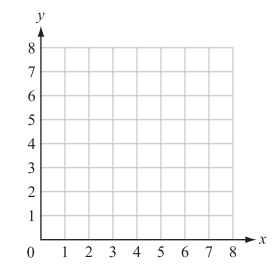Ralph plotted the following points on the coordinate grid:

Point W (2, 1); Point X (2, 6); Point Y (5, 1); Point Z (5, 6)

A polygon is formed with vertices W, X, Y, and Z. Which type of polygon is formed?

Rhombus

Rectangle

Trapezoid

Square

Rectangle

Explanation:

Start by plotting the vertices and connecting them to form a quadrilateral.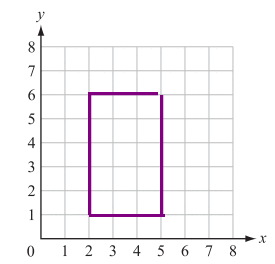The created figure has 4 right angles. This can describe a rhombus, square, and rectangle. Because the sides are not all the same length, the figure must be a rectangle.

### Example Question #2 : Coordinate Geometry

A coordinate plane is shown.Ralph plotted the following points on the coordinate grid:

Point W (0, 0); Point X (7, 0); Point Y (7, 5); Point Z (0, 5)

A polygon is formed with vertices W, X, Y, and Z. Which type of polygon is formed?

Kite

Trapezoid

Triangle

Rectangle

Rectangle

Explanation:

Start by plotting the vertices and connecting them to form a quadrilateral.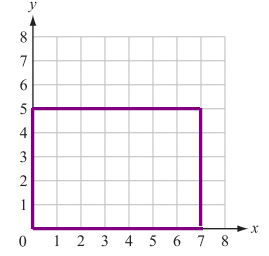The figure that is created has four right angles. Out of the given answer choices, this can only describe a rectangle.

### Example Question #3 : Coordinate Geometry

A shape is plotted on a coordinate plane using the following points: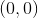,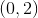,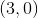, and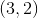. What shape is it?

Square

Trapezoid

Rectangle

Triangle

There is not enough information to determine the shape.

Rectangle

Explanation:

If you plot these points on a coordinate plane, the shape has four sides and opposite sides are equal and parallel, but all sides are not equal.

Therefore, it is a rectangle.

### Example Question #4 : Coordinate Geometry

A shape with points,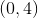,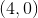, and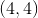is plotted on a coordinate plane. What shape is it?

Trapezoid

Triangle

Prism

Square

Rectangle

Square

Explanation:

Since each side is equal to each other, the shape must be a square.

### Example Question #5 : Coordinate Geometry

A coordinate plane is shown.Ralph plotted the following points on the coordinate grid:

Point W (5, 5); Point X (0, 5); Point Y (0, 0); Point Z (5, 0)

A polygon is formed with vertices W, X, Y, and Z. Which type of polygon is formed?

Kite

Triangle

Square

Trapezoid

Square

Explanation:

Start by plotting and connecting the vertices to create a quadrilateral.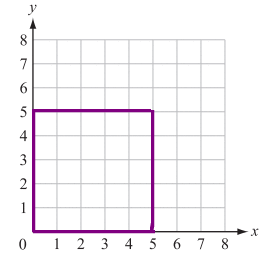The figure that is created has 4 right angles and sides of equal length. The only answer choice that shares these characteristics is the square.

### Example Question #6 : Coordinate Geometry

A square is plotted on a coordinate plane. It includes the following points: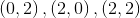.  What is the remaining point?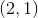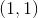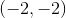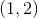Explanation:

Each of the points is written as an ordered pair.  The first number in each ordered pair shows where the point is located on the x-axis.  The second number in each ordered pair shows where the point is located on the y-axis.  If you plot the ordered pairs listed in the problem, the ordered pair that is needed to complete the points of a square is.

### Example Question #7 : Coordinate Geometry

A coordinate plane is shown.Ralph plotted the following points on the coordinate grid:

Point W (3, 3); Point X (3, 8); Point Y (8, 3); Point Z (8, 8)

A polygon is formed with vertices W, X, Y, and Z. Which type of polygon is formed?

Triangle

Pentagon

Trapezoid

Square

Square

Explanation:

Start by plotting and connecting the vertices to create a quadrilateral.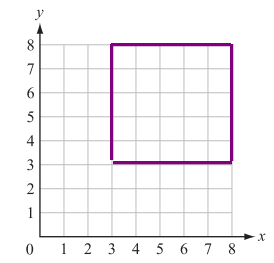The figure that is created has 4 right angles and sides of equal length. The only answer choice that shares these characteristics is the square.

### Example Question #8 : Coordinate Geometry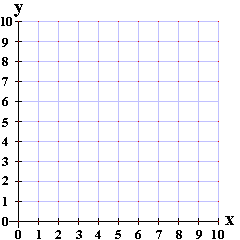Renee plotted the following points in quadrant one on the coordinate grid:

Point A = (2,2)

Point B = (7,2)

Point C= (7,7)

Where in Quadrant one of the coordinate grid would Renee have to plot Point D so that the points form a square with vertices A, B, C, and D, and forms sides AB, BC, CD, and DA?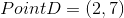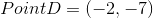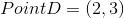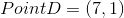Explanation:

Once Points A,B, and C have been plotted the missing coordinates in order to form a square (all sides being equal), Point D would be (2,7). All coordinates (x,y) in Quadrant one will be positive integers.

Another approach is to calculate the distance between each point.

When Point A and Point B are plotted they form a horizontal line that goes from 2 to 7. That makes the distance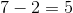.

When Point B and Point C are plotted they form a vertical line that goes from 2 to 7. That makes the distance.

Point C creates a vertical line with Point B.  That makes the distance.

From here, we want to find a point that is horizontally 5 units from Point C and vertically 5 units from Point A. Thus (2,7) is the correct answer.

### Example Question #9 : Coordinate Geometry

A square is plotted on a coordinate plane.  It includes the following points: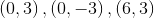What is the remaining point?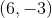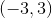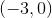Explanation:

Each of the points is written as an ordered pair.  The first number in each ordered pair shows where the point is located on the x-axis.  The second number in each ordered pair shows where the point is located on the y-axis.  If you plot the ordered pairs listed in the problem, the ordered pair that is needed to complete the points of a square is.

### Example Question #10 : Coordinate Geometry

In which quadrant is the point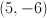located in?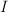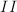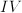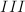From the originis to the right 5 and down 6 making it in quadrant IV.